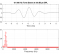## 3D fft MatlabStarted by 7 years ago7 replieslatest reply 7 years ago1724 views

Hello everyone.

I need some advice regarding 3D #FFT on #Matlab. My signal is represented as a 3D matrix (Let's say 10x10x10)

I wanted to find the fft and then the maximum along each dimension.

The way I am doing it is as follows:

signal_fft=fft(signal,[],1)

then [maxAmp Index]= max(abs(signal_fft(:)))

Then using ind2sub to extract x,y,z

I am doing the same for the 2 other dimensions. And i get at the end 3 maximum values and three pairs x,y,z that are not the same.

If I would like to then extract the frequency out of this indices how should I do it?

e.g: 1D normally  I get one Index after computing max. and then f_Index=(Index-1)*Fs/length(signal)

Any reply would be greatly appreciated

[ - ]I always screw up my kids when I try to help with their MATLAB homework, so hopefully somebody else here can help with that part.  But it sounds like you are not doing a 3D FFT on your 3D data set.  If you have a 2D plane, you do an FFT for each row and each column.  For 3D, you will need to perform an FFT for each row and column in each plane, and then an FFT in the perpendicular direction for each entry in the plane.  So in the end, for your 10x10x10 example, you need to do 1000 FFT's.  Then you compute the magnitude, and then you search for the highest value in the volume.

There is a lot that can go wrong, so start with 2D and make sure you can do a plane first, then go to 3D.

Mike

[ - ]Hello,

I agree with Mike.  Start with 2D cases and work your way to 3D.  Although I've done a bit of work using FFTs, it's been primarily with speech signals.  Adding extra dimensions makes the problem more difficult to comprehend and trouble-shoot if the results do not meet expectations.

To address the issue of knowing whether or not your code works as expected, I would suggest using test cases.

[ - ]To clarify what Mike said (if it's needed): you do an FFT on each row in each plane, making a new 3D matrix.  Then you do an FFT on each column in each plane of that once-FFT'd data, making another new 3D matrix (and possibly discarding your last intermediate result).  Then, finally, you do yet another FFT across all the planes on that twice-FFT'd data, getting your 3D result.

I don't know how Matlab defines their fft, but the most likely thing they'll do with a 2D matrix is to make a 2D FFT (i.e., the first two steps that I outline above), leaving you to do the across-plane FFTs.  If the Matlab 3D matrix syntax allows you to extract a vector across planes then you can do those 100 vectors in a for loop.

Doing this in a compact manner is left as an exercise for the Matlab whiz -- I don't even know how to do it in Scilab, which is my chosen numerical analysis tool.

[ - ]If I may add: putting a 3D matrix of data into the frequency domain seems odd.  I'm sure there are problems where it's the right thing to do -- but if you haven't done so already, you may want to ask yourself _why_ you're doing it.

[ - ]Hmm, maybe for 3D you should consider moving to an AI platform such as Google's Open Source TensorFlow.

[ - ]£D fft is not a problem in Matlab, here is how Matlab suggests:

X = randn(5,5,5);
Y = X;
for p = 1:length(size(X))
Y = fft(Y,[],p);
end

The OP is not having difficulty about fft3 but rather is asking about frequency at maximum amplitude of fft outputs. I don't myself know what 3D frequency domain means but I would say it is about rate of change of values across each plane and a final value can be the max of all maximum values indicating highest content at that point of frequency. The actual translation to absolute frequency may then be computed as for 1D case. Just a thought.

Kaz

[ - ][maxAmp, Index] = max(abs(signal_fftn(:)))[x,y,z] = ind2sub(size(signal_fftn), Index)